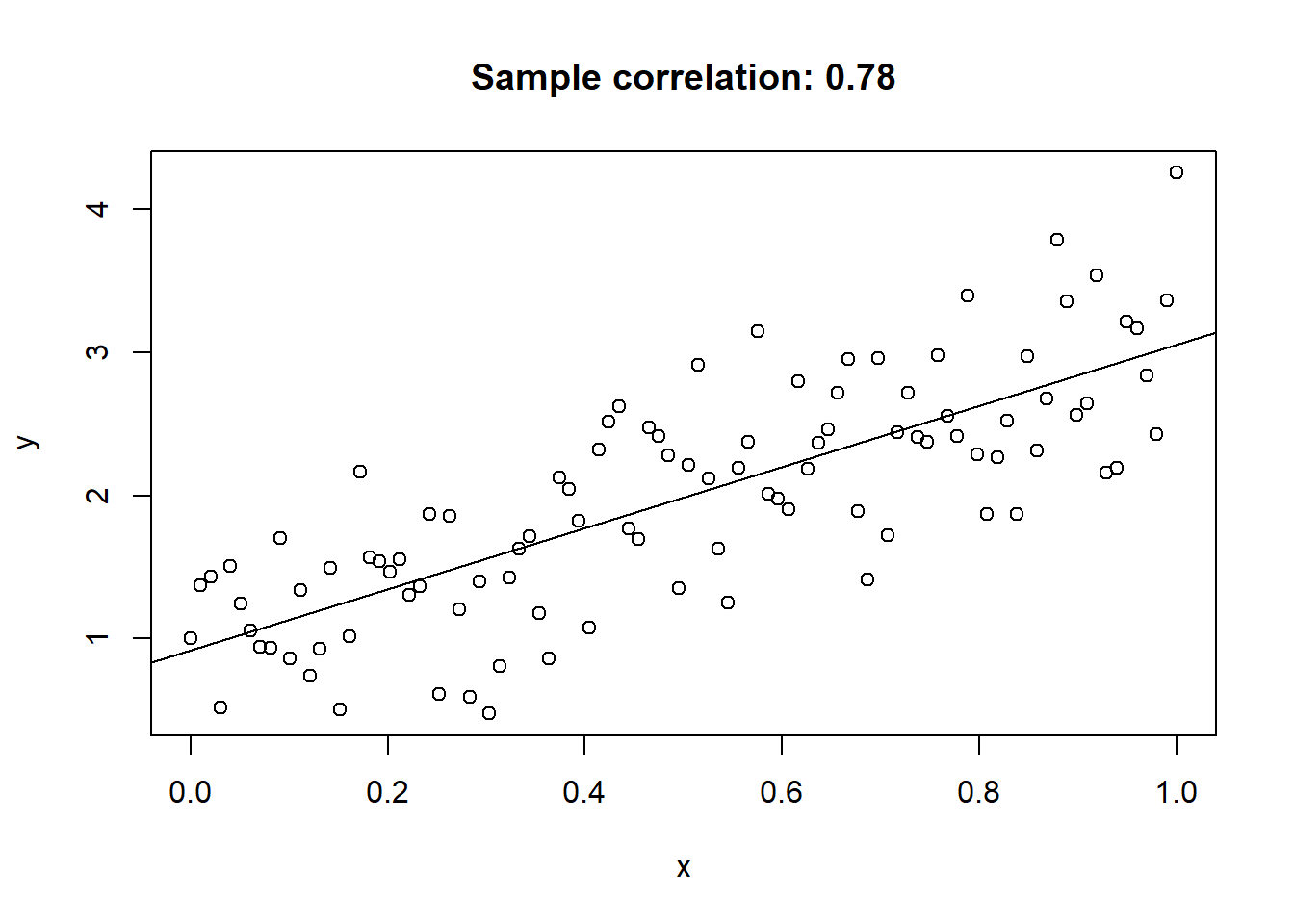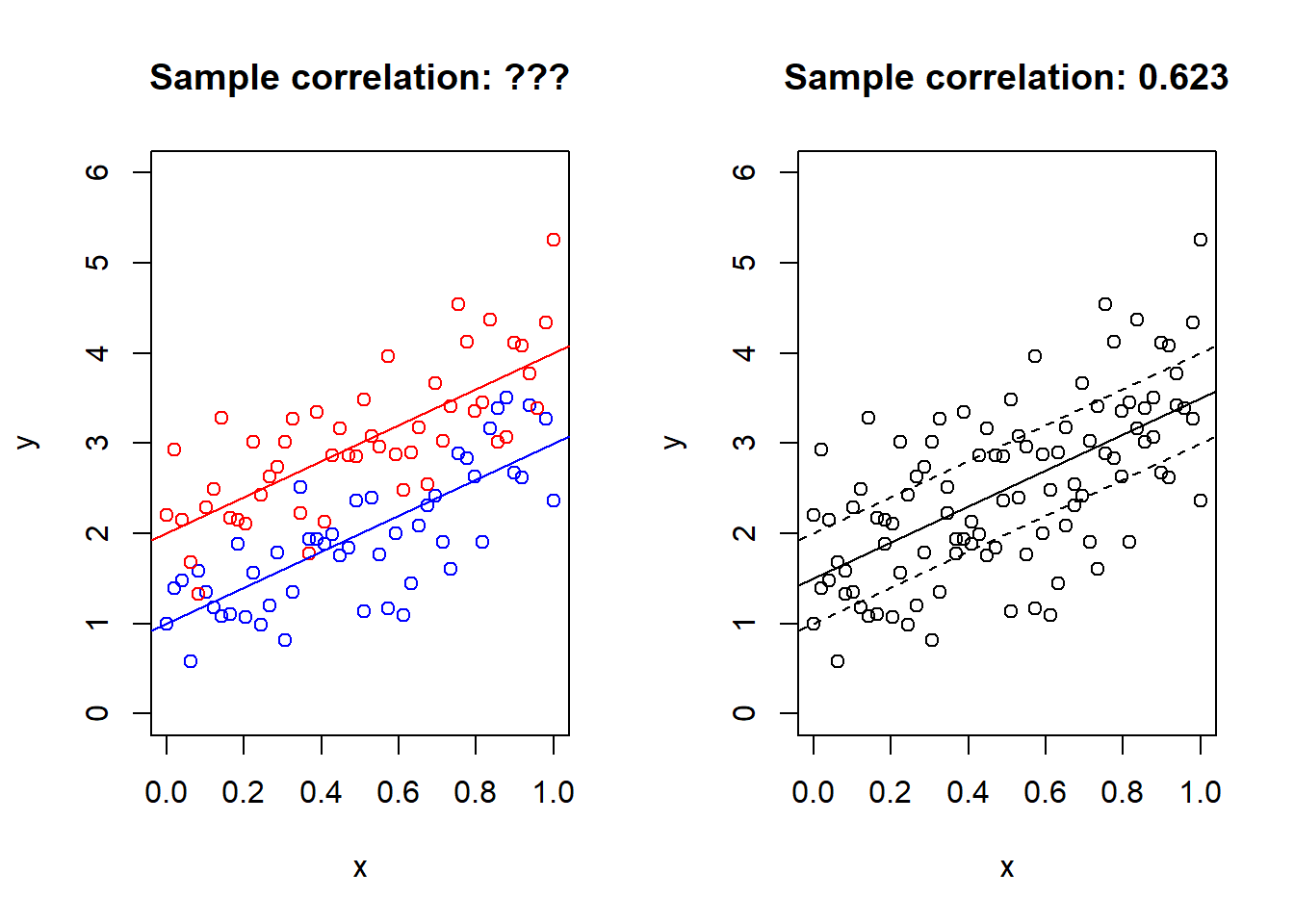# Correlation with two lines!

statistics
joking
Author

Jonas Moss

Published

Jan 25, 2023

Everyone knows the correlation coefficient. It measures the degree of linear dependence between two random variables $$X$$ and $$Y$$. It finds the best-fitting line $$Y=a+bX$$ and measures how far the observed values of $$Y$$ are from the predicted values of $$y$$ given $$X$$.

Generate and plot some data from a line.
set.seed(313)
n = 100
x = seq(0, 1, length.out = n)
y = 2 * x + 1 + rnorm(n) * 0.5
plot(x, y, main = paste0("Sample correlation: ", round(cor(x,y), 3)))
abline(lm(y ~ x))Plot of data simulated from $$y = 2x+1$$.

That should be familiar. But what if there are TWO parallel lines in the data?!

Generate ad plot some data frome two lines!
set.seed(313)
par(mfrow=c(1,2))
n = 50
x1 = seq(0, 1, length.out = n)
y1 = 2 * x1 + 1 + rnorm(n) * 0.5
x2 = seq(0, 1, length.out = n)
y2 = 2 * x2 + 2 + rnorm(n) * 0.5
plot(x1, y1, main = paste0("Sample correlation: ???"), col = "blue", ylim = c(0, 6), xlab = "x", ylab = "y")
points(x2, y2, col = "red")
abline(a = 1, b = 2, col = "blue")
abline(a = 2, b = 2, col = "red")
x = c(x1, x2)
y = c(y1, y2)
z = c(rep(0, 50), rep(1, 50))
plot(x, y, main = paste0("Sample correlation: ", round(cor(x,y), 3)), xlab = "x", ylab = "y", ylim = c(0, 6))
abline(a = 3/2, b = 2)
abline(a = 1, b = 2, lty = 2)
abline(a = 2, b = 2, lty = 2)Plot of data simulated from $$y = 2x+1$$ and $$y = 2x+2$$!

The data has been simulated from the model $\begin{eqnarray*} y\mid x,z=0 & \sim & 2x+1+\frac{1}{2}\epsilon,\\ y\mid x,z=1 & \sim & 2x+2+\frac{1}{2}\epsilon. \end{eqnarray*}$ where $$z$$ is a group indicator and $$\epsilon$$ is standard normal. Moreover, the $$x$$s are $$50$$ observations uniformly spaced on $$[0,1]$$. What is the marginal model, with $$z$$ integrated out? It’s $$2x + 3/2$$, displayed on the right. The plot on the right certainly doesn’t look like it contains two lines.

If we know the joint distribution of $$(X,Y,Z)$$ , generalizing the correlation is easy! The ordinary correlation coefficient can be written as $$\operatorname{Cor}(X,Y)=\operatorname{sign}\beta\sqrt{R^{2}}$$,where $R^{2}=1-\frac{\min_{a,b}E\left[(Y-a-bX)^{2}\right]}{\min_{\mu}E\left[(Y-\mu)^{2}\right]},$ and $$\beta$$ is the minimizing slope of the numerator. Now just add the covariate $$1[Z=1]$$ to the linear regression model in the denominator, and the resulting correlation becomes $\operatorname{Cor}(X,Y;Z)=\operatorname{sign}\beta\sqrt{R_\star^{2}},$ where $R^{2}_\star=1-\frac{\min_{a,b}E\left[(Y-a_{0}-a_{1}1[Z=1]-bX)^{2}\right]}{\min_{\mu}E\left[(Y-\mu)^{2}\right]},$ and $$\beta$$ is the minimizing slope of the numerator (the sign of the slope is unambiguous since we’ve assumed the lines are parallel).

Calculating this correlation coefficient is easy as pie.

mod = lm(y ~ x + z)
unname(sqrt(summary(mod)$r.sq) * sign(coef(mod)))  0.8495882 And its much larger than the “one-line correlation”, going from $$0.62$$ to $$0.85$$. But we can’t calculate it without knowing $$Z$$! ## Defining a correlation for two parallel lines So, we know the joint distribution of $$(x,y)$$, and nothing more. How can think about the “two-lines correlation”? I can only think of one reasonable way to solve the problem. We need to find lower and upper bounds for $$\operatorname{Cor}(X,Y;Z)$$. Because we might not know $$Z$$, but maybe we can find bounds for the correlation $$\operatorname{Cor}(X,Y;Z)$$ that we could have calculate had we known $$Z$$. ### Finding bounds I can’t think of an efficient way to calculate upper bounds, but the lower bound is simple enough (in the case when the correlation is positive): It equals $$\operatorname{Cor}(X,Y)$$. When dealing with estimation problems, a reasonable estimator of the upper bound would be to make every possible split of the data into into two categories (according to z)and fit the regression model y ~ x + z. But that would be $$2^n$$ combinations, which is obviously intractable for large $$n$$. It might be possible to find exact algorithms that aren’t exponential in time, indeed, I would expect it, but I can’t justify spending more time on this problem. However, consider the following data n <- 15 x <- mtcars$drat[1:n]
y <- mtcars$wt[1:n] Since $$2^{15}= 32768$$ we don’t need to fit that many linear regressions to estimate the upper correlation bound in this case. Code estimating a bunch of regression models. n <- 15 x <- mtcars$drat[1:n]
y <- mtcars$wt[1:n] n <- length(x) mat <- cbind(1, x, 0) minimum = Inf for(k in seq(0:n)) { icomb <- arrangements::icombinations(n, k) choose(n, k) for(i in seq(choose(n, k))) { indices <- icomb$getnext()
mat[, 3] <- 0
mat[indices, 3] <- 1
mod <- lm.fit(mat, y)
current <- sum(mod$residuals^2) if (current < minimum) { minimum = current z = indices coef <- mod$coefficients
}
}
}

cor2 <- sqrt(1 - minimum/sum((y - mean(y))^2)) * sign(coef)
cor1 <- cor(x, y)

From the code above we find that lower and upper bounds for the two lines correlation:

c(lower = cor2, upper = cor1)
   lower.x      upper
-0.8834406 -0.6358763 

Let’s also have a look at the classification of points that maximizes this correlation.

Code for plot.
col <- rep("blue", n)
col[z] <- "red"
plot(x, y, col = col, xlab = "Rear axle ratio", ylab = "Weight",
pch = 20, main = "Two lines or one line?")
abline(a = coef, b = coef, col = "blue")
abline(a = coef + coef, b = coef, col = "red")
abline(lm(y ~ x))